# Fundamentals of Engineering Economics

## Quiz 2 : Time Value of MoneyStudy FlashcardsLooking for Economics Homework Help?

## Quiz 2 :Time Value of Money

Question TypeCompare the interest earned on $15,000 for 25 years at 7% simple interest with the amount of interest earned if interest were compounded annually. Free Essay Answer: Answer: Given information: • Amount deposited is$15,000.
• At 7 percent simple interest rate.• Time period is 25 year.
• At 7 percent compound interest rate.The total amount of interest earned from present worth of deposit:
The total amount of interest earned from present worth of deposit can be calculated by using the following formula: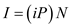…… (1)Where,
I = Total amount of interest rate earned
P = Present value
N = Number of years
i = Simple rate of interest
Substitute the respective values in Equation (1), to obtain the total amount of interest earned from the present worth of deposit.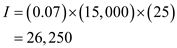Hence, the total amount of interest earned from the present worth of deposit is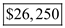.
The total amount of interest earned from present worth of deposit:
The total amount of interest earned from the present worth of deposit can be calculated by using the following formula: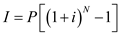…… (2)Where,
I = Total amount of interest rate earned
P = Present value
N = Number of years
i = Compound rate of interest
Substitute the respective value in Equation (2), to obtain the total amount of interest earned from the present worth of deposit.Hence, the total amount of interest earned from the present worth of deposit is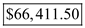.

Tags
Choose question tagWhat is the amount of five equal annual deposits that can provide five annual withdrawals, where a first withdrawal of $1,000 is made at the end of year 6 and subsequent withdrawals increase at the rate of 10% year over the previous year's if the interest rate is 10% compounded annually? (a)$745 (b) $789 (c)$1,000 (d) $1,563 Free Multiple Choice Answer: Answer: Given information: • Five annual withdrawals. • First withdrawal at the end of year 6 is$1,000.
• Interest rate compounded annually is 10 %.
• Annual cash withdrawals are expected to increase at the rate of 10 %.
In order to obtain the amount of equal annual deposit for the first 5 years, the future equivalent worth of annual cash deposit for first 5 years (0-5) is set equal to the to present equivalent worth of annual cash withdrawals for the next 5 years (6-10).
Amount of equal annual deposit for the first 5 years:
Amount of equal annual deposit for the first 5 years can be obtained by using the following formula:…… (1)Where,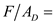Future equivalent worth of annual cash depositPresent equivalent worth of annual cash withdrawals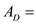Annual cash deposit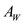= Annual cash withdrawals
i = Interest rate
N = Number of years
Substitute the respective values in Equation (1) to obtain the amount of equal annual deposit for the first 5 years.Since the amount of equal annual deposit for the first 5 years is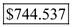, option a is correct.

Tags
Choose question tagWhat value of C makes these two cash flows equivalent at an interest rate of 10%?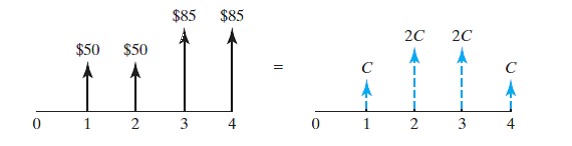(a) $29.65 (b)$35.98 (c) $47.33 (d)$43.96
Free
Multiple Choice

Given information:
Table-1 shows the cash flow of the two projects A and b.Table-1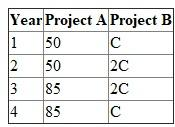• Interest rate is 10 %.
Value of C:
Value of C can be calculated by using the following formula:…… (1)Where,
n = time period
I = interest rate on year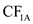= cash flow of project A for year 1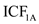= increasing cash flow of project A from year 1
Substitute the respective values in Equation (1) to calculate the value of c.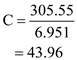The value of C is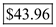. Hence, option d is correct.

Tags
Choose question tagFour years ago, you opened a mutual fund account and made three deposits ($200 four years ago,$X three years ago, and $300 a year ago) where you earned varying interest rates according to the following diagram. Today, your balance shows$1,000. Determine the amount of deposit that was made three years ago ($X). See the following figure. (a)$215 (b) $237 (c)$244 (d) $259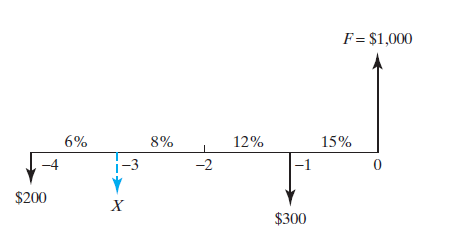Multiple Choice Answer: Tags Choose question tagYou wish to have$10,000 in an account 10 years from now. How much money must be deposited in the account now in order to have this amount if the account pays 8% compounded annually? (a) $1,490 (b)$3,871 (c) $4,632 (d)$5,584
Multiple Choice
Tags
Choose question tagYou deposit $3,000 in a savings account that earns 8% simple interest per year. How many years will it take to double your balance? If, instead, you deposit the$3,000 in another savings account that earns 7% interest compounded yearly, how many years will it take to double your balance?
Essay
Tags
Choose question tagYou borrow $20,000 from a bank to be repaid in three equal annual installments at 9% interest compounded annually. What is the portion of interest payment for the second annual payment? (a)$1,800 (b) $1,251 (c)$1,089 (d) $549 Multiple Choice Answer: Tags Choose question tagThe following two cash flows are said to be economically equivalent at 10% interest. Determine the value of X for the second cash flow series.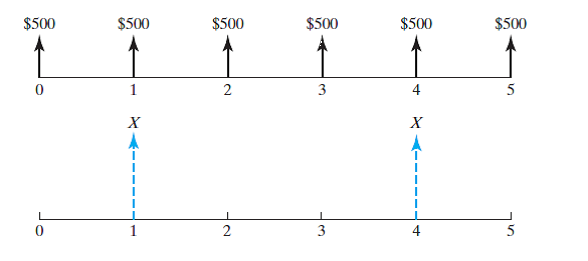(a) X =$1,505 (b) X = $1,500 (c) X =$1,197 (d) X = $1,192 Multiple Choice Answer: Tags Choose question tagWhat single payment at the end of year 5 is equivalent to an equal annual series of payments of$800 beginning at the end of year 3 and ending at the end of year 12? The interest rate is 8% compounded annually. (a) $5,797 (b)$6,260 (c) $6,762 (d)$6,883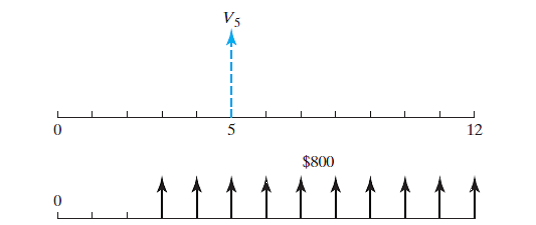Multiple Choice
Tags
Choose question tagWhat is the amount of interest earned on $2,000 for five years at 6% simple interest per year? Essay Answer: Tags Choose question tagWhich of the following alternatives would you choose, assuming an interest rate of 10% compounded annually? Alternative 1: Receive$100 today. Alternative 2: Receive $120 two years from now. Alternative 3: Receive$170 five years from now.
Essay
Tags
Choose question tagCalculate the future worth of 20 annual $2,000 deposits in a savings account that earns 9% (compounded annually). Assume that all deposits are made at the beginning of each year. (a)$126,005 (b) $111,529 (c)$113,529 (d) $92,037 Multiple Choice Answer: Tags Choose question tagAssume that$500 is deposited today, two years from now, four years from now, six years from now, and eight years from now. At a 10% interest compounded annually, determine the future value at the end of year 9.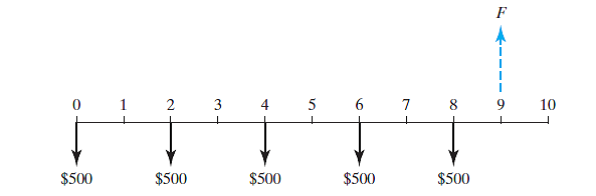(a) $4,174 (b)$3,790 (c) $2,085 (d)$1,895
Multiple Choice
Tags
Choose question tagToday you deposited $1,000 in a savings account paying 5% annual interest. How much should you have at the end of three years? Essay Answer: Tags Choose question tagHow much money should be deposited now in an account that pays 10% interest compounded annually in order to make five equal annual withdrawals of$5,000? (a) $8,052 (b)$9,050 (c) $16,761 (d)$18,954
Multiple Choice
Tags
Choose question tagBank A pays 6% simple interest on its savings account balances. Bank B pays 5.5% interest compounded annually. If you made a $10,000 deposit in each bank, which bank provides you more money at the end of 15 years? Essay Answer: Tags Choose question tagSuppose that, to cover some of your college expenses, you are obtaining a personal loan from your uncle in the amount of$25,000 (now) to be repaid in two years. If your uncle could earn 8% interest (compounded annually) on his money invested in various sources, what minimum lump-sum payment two years from now would make your uncle happy economically?
Essay
Tags
Choose question tagSuppose you have the alternative of receiving either $5,000 at the end of five years or P dollars today. Currently, you have no need for the money, so you could deposit the P dollars into a bank account that pays 7% interest compounded annually. What value of P would make you indifferent in your choice between P dollars today and the promise of$5,000 at the end of five years?
Essay
Tags
Choose question tagYou are considering investing $1,000 at an interest rate of 6.5% compounded annually for five years or investing the$1,000 at 6.8% per year simple interest for five years. Which option is better?
EssayYou are about to borrow $10,000 from a bank at an interest rate of 9% compounded annually. You are required to make five equal annual repayments in the amount of$2,570.92 per year, with the first repayment occurring at the end of year 1. For each year, show the interest payment and principal payment.